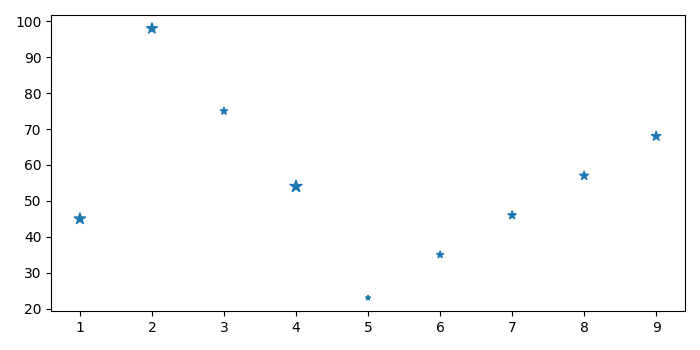# How to modify a 2d Scatterplot to display color based on a third array in a CSV file?

MatplotlibPythonData Visualization

To modify a 2d scatterplot to display color based on a third array in a CSV file, we can take the following steps −

• Set the figure size and adjust the padding between and around the subplots.
• Create a new figure or activate an existing figure.
• Add an 'ax' to the figure as part of a subplot arrangement.
• Make a scatter plot with CSV file data points.
• To display the figure, use show() method.

## Example

import pandas as pd
from matplotlib import pyplot as plt

plt.rcParams["figure.figsize"] = [7.00, 3.50]
plt.rcParams["figure.autolayout"] = True

columns = ["data1", "data2", "data3"]

fig = plt.figure()
ax.scatter(df.data1, df.data2, df.data3, marker="*")

plt.show()

The "input.csv" file contains the following data

data1data2data3
14571
29865
37529
45463
52312
63527
74639
85744
96851

## Output

When we run the code, it will produce the following scatter plot